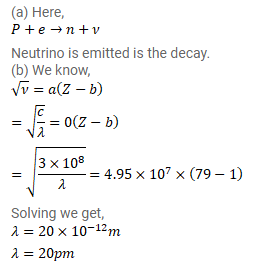# 80197Hg decays to 79197Au through electron capture with a decay constant of 0.257 per day.

Question:

$80197 \mathrm{Hg}$ decays to 79197Au through electron capture with a decay constant of $0.257$ per day.

(a) What other particle or particles are emitted in the decay?

(b) assume that the electron is captured from the $\mathrm{K}$ shell. Use moseley's law

Solution: